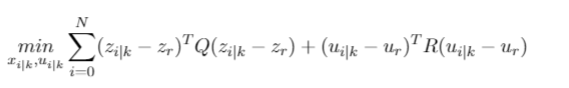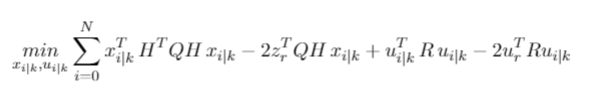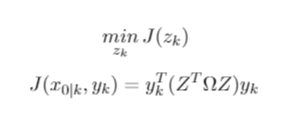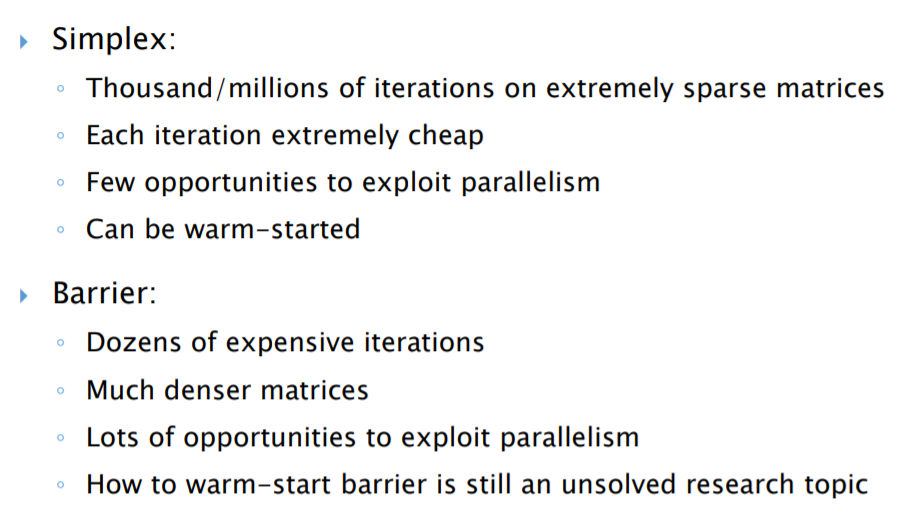•Gurobi Staff

You should really consider re-formulating your model, as the reported ranges, especially for the quadratic,

> Matrix range [2e-09, 6e+01]
> Objective range [8e-04, 5e+03]
> QObjective range [2e-09, 1e+05]

border-line with the round-off error in double-precision arithmetic (think on any "standard" PC if you try this logical compassion, (1 + 1e-16 == 1), you will get TRUE).  This subject is covered in details in our online doc,

https://www.gurobi.com/documentation/8.1/refman/numerics_gurobi_guidelines.html

and as a first thing one can try, even a simple re-scaling of the variables often helps. For instance, to avoid large quadratic entries on the (I,i) diagonal you can change the meaning of your variable where the respective x_i would count things not in units, but in thousand of units.  For more details, please read through the above manual online.

Hope this helps,

•Hello Yuriry,

Does the QObjective range specify the range of the elements in the Hessian? Is is somewhat the same as the condition number of the Hessian?

The right-hand side of the inequality constraint is a vector which contains the values 0 and 10, which represents the bounds of the values the decision variables can take. So, I think the right units are chosen to express the variables and constraints.

Furthermore, I don't really understand the proposed possible solution: '' to avoid large quadratic entries on the (I,i) diagonal you can change the meaning of your variable where the respective x_i would count things not in units, but in thousand of units''.

I have read the manual, but I cannot really find this back. What do you mean with (l,i) diagonal and x_i for example?

Regards

•Gurobi Staff

QObjective range specifies the range of the matrix in the quadratic objective, and you can think of it as the Hessian of the objective as well.  The right hand side of the constraints is not necessarily the best reference point -- it is much more important to keep the ranges of 'matrices' (objective and constraint coefficients) in check.

"To avoid large quadratic entries on the (i,i) diagonal you can change the meaning of your variable where the respective x_i would count things not in units, but in thousand of units''.

Suppose your objective has a term

a(i,i) * x(i)^2

where a(i,i) is very small, say on the order of 10^(-9).  Then by changing the respective x(i) to represent 10^(-4)*x(i) units, say re-writing the model in terms of y(i) = 10^(-4) x(i), you can replace the

a(i,i) * x(i)^2

term by

(a(i,i) * 10^8) * y(i)^2

effectively scaling the diagonal entry of your quadratic objective matrix by 10^8 (so the new coefficient will be on the order of 10^(-1)).

Hope this helps.

•Hi,

Thanks for the clarification.

Part 1:

The optimization problem I am solving could be seen below:with zi|k = H*xi|k, ui|k the predicted inputs, xi|k the predicted statesIn this optimal control problem Q and R are both symmetric positive definite matrices, with Q a diagonal matrix (all diagonal entries have the value 100) and R a diagonal matrix (all diagonal entries have the value 0.1). Furthermore, the mean of the vector of z_r is around 5 and the mean of the vector u_r is around 0.1.

•Part 2:

In more detail, after some substitution the quadritic part of the optimization problem looks like:with Z a banded null-space matrix, Omega a diagonal matrix with transpose(H)*Q*H  and R on the diagonal entries.

The condition number of Z is 1e7. Therefore, I think the problem lays in the Z matrix.

Is it for example possible to use your example of re-scaling for the term transpose(Z)*Omega*Z? If yes, what should be done with the linear objective term which is not displayed above? Or is there in this case a better possible solution?

Regards

•Gurobi Staff

Thanks for providing more details, and yes, I would try the re-scaling that was described earlier to see what happens.  For the linear term, I would apply the same change of variable and not worry (at least at first) about the effect on the linear term; usually the quadratic is the one causing numerical problems.  If you see that the linear part becomes problematic, you can consider using a less-aggressive scaling as well.

Cheers,

•Hi,

I have re-scaled the model, but the numerical issues are still present.

Do you have any other options I could try?

What did you by the way mean with: border-line with the round-off error in double-precision arithmetic (think on any "standard" PC if you try this logical compassion, (1 + 1e-16 == 1), you will get TRUE)?

That Matlab makes round-off errors due to floating point errors?

And do you have maybe a possible explanation why the numerical issues for Gurobi occur and not for Matlabs quadprog function (or at least for very large matrices)? Both are making use of the barrier/interior-point method.

Kind regards,

•Gurobi Staff

This subject is covered in details in our online doc,

https://www.gurobi.com/documentation/8.1/refman/numerics_gurobi_guidelines.html

> .. floating point errors?

yes, flowing point arithmetic errors will be persistent on any platform, and not limited to Matlab.

I cannot tell what is going on in Matlab quadprog, but it is possible that some issues are circumvented there, and it is also possible that these things are simply not reported and ignored.   I would certainly doble-check the answers as part of post-processing the solution, to make sure the solution is feasible etc.

Hope this helps.

•Hi,

Thanks for your response. Still one general question about Gurobi (you maybe know from experience).

I have some general questions about the barrier algoritm that Gurobi uses to solve a QP.

For which type of problems does the algorithm work the best? Large-scale systems or small-scale systems?

Do dense matrices work the best for the barrier algorithm or sparse matrices? What are the causes of that? Or works a combination better?

For example small,  the fastest for small, dense problems and large, sparse problems.

Does the algorithm need a lot of iterations to converge or less iterations, but each iteration is more expensive, in general?

I found a Powerpoint about Gurobi and this was the only thing I found of the above subject, but I don't exactly know what they mean with:

Dozens of expensive iterations: Why many iterations and expensive at both time

Much denser matrices: Why denser matrices? I thought Gurobi could handle sparse matrices better?

Lots of opportunities to exploit parallelism: What is parallelism?